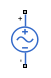# SFFM Voltage Source

Single-frequency frequency-modulated voltage source

•Libraries:
Simscape / Electrical / Additional Components / SPICE Sources

## Description

The SFFM Voltage Source block represents a frequency-modulated AC voltage source whose output voltage value is independent of the current through the source. The following equation describes the output voltage as a function of time:

`${V}_{out}=VO+VA*\mathrm{sin}\left(\left(2\pi *FC*Time\right)+MI*\mathrm{sin}\left(2\pi *FS*Time\right)\right)$`

where:

• V0 is the Voltage offset, VO parameter value.

• VA is the Voltage amplitude, VA parameter value.

• FC is the Carrier frequency, FC parameter value.

• MI is the Modulation index, MI parameter value.

• FS is the Signal frequency, FS parameter value.

## Ports

### Conserving

expand all

Electrical conserving port associated with the SFFM voltage source positive voltage

Electrical conserving port associated with the SFFM voltage source negative voltage

## Parameters

expand all

Magnitude of the time-independent part of the output voltage.

Magnitude of the sinusoidal part of the output voltage.

Frequency of the carrier wave. The value must be greater than or equal to `0`.

Amount by which the modulated signal varies around its unmodulated level. The value must be greater than or equal to `0`.

Frequency of the modulated signal. The value must be greater than or equal to `0`.

## Version History

Introduced in R2008a